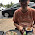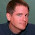### All we need is a really long tether!

I've written previously about non-rotating artificial gravity in Earth orbit. After recently watching this stinker I broke out the code I use to figure out gravity gradient effects. Surprisingly, this seems pretty good:

AltitudeMassGravity
LEO Station300 km273 tons0.999 g
GEO Station35786 km30 tons0.38 g

Of course, this is a much longer tether than in the film.. but hey, Danny Baldwin is in it - he doesn't make good movies. Anyway, the high station could be an ISS-style module with airlock and docking ports for satellite servicing vehicles. The low station would be a true permanently inhabited facility where people can live for years at a time without fear of bone mass deterioration or the other negative effects of zero gravity. To maximize space we may be tempted to use inflatable Bigelow modules, but we have to consider how they will behave in full gravity.

The only sticking point left is radiation. On the LEO station crews have much less exposure to cosmic radiation thanks to the Earth's magnetic field, however they receive just as much from flying through the South Atlantic Anomaly. As a result, radiation on the GEO station would be 2.19 times as high during solar maximum and 6.568 times as high during solar minimum. If that seems confusing, just remember that the Sun's magnetic field provides most of our protection against cosmic radiation, and it does that more at maximum than at minimum.

One solution may be minimagnetospheres but, again, technology developed for zero-g rarely works unmodified in full gravity. The best solution may simply be appropriate mass. The requirement that the low station be 9.1 times more massive than the high station means that both will have to grow simultaneously but getting mass from LEO to GEO is pretty easy when you have a tether joining the two altitudes.

You may be asking: how plausible is this? Or even: isn't this just the Space Elevator? I estimate it is at least two orders of magnitude easier to do than a space elevator and would only require (vast amounts of) existing tether materials. The cost is most likely dominated by launch costs that should be around \$800M in a few years time.

1."You may be asking: how plausible is this?"

No, rather "how practical?" Why use such a long tether when shorter and far lighter rotating tethers would be cheaper, work better for most applications, aren't as vulnerable to meteoroid damage, and don't pose as great a hazard to other in space activities?

2.3.Andrew, ya just hafta understand. Trent plans on building a Dyson Sphere... one tether at a time!

0.38g at GEO is a sign... the next step mars (or mercury.)

4.haha.. yes, the 0.38g was why I found it interesting.. and as far as I can tell, it's a total coincidence.

5.What does the orbit of the LEO station look like? At an effective .999g I assume it's not going very fast. If so, that would take some of the sting out the cost of "reaching orbit", turning it into an incremental step over sub-orbital flights.

(EDIT: stupid typo.)

6.Trent, what's the orbital period of this proposed tether? 0.999g at 300km suggests very long (24 hours?), but 0.38 at GEO suggests very short (90 minutes?).

7.The center of mass is at an altitude of 3813.5 km, so the orbital velocity would be 6.25 km/s and the orbital period would be just under 171 minutes. So it's a little bit of a win on the LEO end but means you're going much too fast on the GEO end :(

8..999g from a 171-minute orbit? That doesn't make sense at the LEO end. I was expecting something a lot closer to 24 hours to get such a high percentage of surface G. What am I missing here?

9.If r = earth_radii + lower_altitude
and the center of mass of the tether is l = upper_mass / (lower_mass + upper_mass) * tether_length
Then the gravity at the low end is g = -earth_gm / ((r + l) * (r + l)) + earth_gm / (r*r*r) * (r + l)
Similarly if r2 = r + tether_length
Then the gravity at the high end is g2 = -earth_gm / ((r + l) * (r + l)) + earth_gm / (r2*r2*r2) * (r + l)

The constants are: earth_radii = 6378.14, earth_gm = 398600.436, and divide g and g2 by 0.00981 to get proportions of Earth gravity. All lengths in km. All masses in kg.

10.Trent, I get an angular velocity .00061 radians/second.

Acceleration from so called centrifugal force is = w^2 * r. Picking r = 6678 kilometers, acceleration is about 2.5 meters/second^2.

At 6678 I get acceleration from earth's gravity is 8.9 meters/second^2.

8.9 - 2.5 = 6.4. I get a net acceleration of 6.4 m/s^2 or about 2/3 of a g.